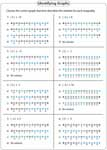Absolute Value Inequalities Worksheets

There are 45 absolute value inequalities worksheets in this page. Convert each absolute value inequalities into two simple linear inequalities and solve the problems. 'No solution' cases also included. Students must be careful about extraneous solutions.

Solving Absolute Value Inequalities

Solving absolute value inequalities worksheets contain 12 problems in basic types and 10 problems in both one-step and two-step.Basic:

One-step:

Two-step:

Solving and Graphing Inequalities: Standard

Best practice worksheets that reinforce the knowledge in solving and graphing absolute value inequalities.Basic:

One-step:

Two-step:

Solving and Graphing: Multiple Choices

Solve each absolute value inequality and find the correct graph that best describes the solution.Basic:

One-step:

Two-step:

Multiple Choices: Standard

The question type is based on multiple choices. Solve and find the correct solution.Basic:

One-step:

Two-step:

Multiple Choices: Interval Notation

The worksheets contain multiple choices based on solving inequalities with solutions in interval notation.Basic:

One-step:

Two-step:

Develop skills with the entire collection of worksheets on absolute value inequalities.

Related Worksheets